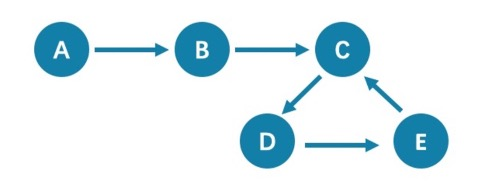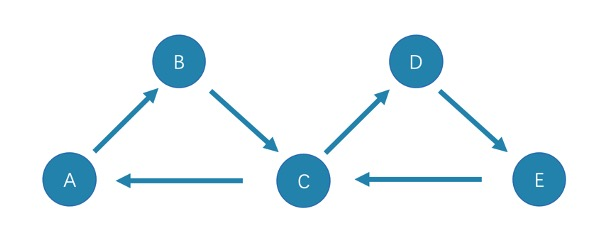# Path types¶

In graph theory, a path in a graph is a finite or infinite sequence of edges which joins a sequence of vertices. Paths are fundamental concepts of graph theory.

Paths can be categorized into 3 types: walk, trail, and path. For more information, see Wikipedia.

The following picture is an example for a brief introduction.## Walk¶

A walk is a finite or infinite sequence of edges. Both vertices and edges can be repeatedly visited in graph traversal.

In the above picture C, D, and E form a cycle. So, this picture contains infinite paths, such as A->B->C->D->E, A->B->C->D->E->C, and A->B->C->D->E->C->D.

Note

GO statements use walk.

## Trail¶

A trail is a finite sequence of edges. Only vertices can be repeatedly visited in graph traversal. The Seven Bridges of Königsberg is a typical trail.

In the above picture, edges cannot be repeatedly visited. So, this picture contains finite paths. The longest path in this picture consists of 5 edges: A->B->C->D->E->C.

Note

MATCH, FIND PATH, and GET SUBGRAPH statements use trail.

There are two special cases of trail, cycle, and circuit. The following picture is an example for a brief introduction.• cycle

A cycle refers to a closed trail. Only the terminal vertices can be repeatedly visited. The longest path in this picture consists of 3 edges: A->B->C->A or C->D->E->C.

• circuit

A circuit refers to a closed trail. Edges cannot be repeatedly visited in graph traversal. Apart from the terminal vertices, other vertices can also be repeatedly visited. The longest path in this picture: A->B->C->D->E->C->A.

## Path¶

A path is a finite sequence of edges. Neither vertices nor edges can be repeatedly visited in graph traversal.

So, the above picture contains finite paths. The longest path in this picture consists of 4 edges: A->B->C->D->E.

Last update: September 2, 2021